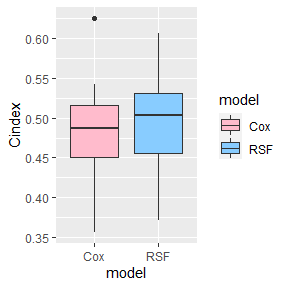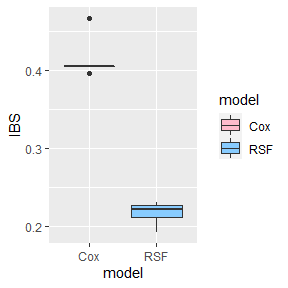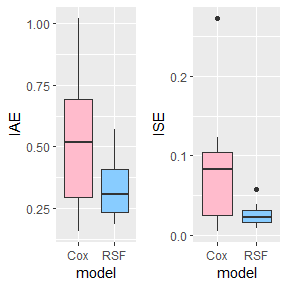# Vignette

## 1. Introduction

Survival analysis aims to study the relationship between independent covariates and survival time and event outcomes. With the increasing demand for accuracy in practical applications, survival models are constantly being developed and improved. Meanwhile, how to measure the prediction performance of survival models has also attracted more and more attention (). The censoring problem of survival data is the main reason why survival models are difficult to evaluate (; ). In this package, we want to provide an “all-in-one” package which includes most of the evaluation metrics for survival predictions.

In the following, we will show how these model evaluation metrics work based on some simulated datasets and the popular Cox and random survival forest (RSF) models.

## 2. Data

The four simulated survival data scenarios used here have different degrees of violation of the proportional hazard assumption and are constructed as follows:

Dataset 1: Data is created using 300 independent observations, where the covariate vector $$( W_1, . . . ,W_{25} )$$ is multivariate normal with mean zero and a covariance matrix having elements $$(i, j)$$ equal to $$0.9^{|i−j|}$$. Survival times are simulated from an exponential distribution with mean $$\mu = e^{0.1 \sum_{i=11}^{20}} W_i$$ (i.e., a proportional hazards model) and the censoring distribution is exponential with mean chosen to get approximately 30% censoring.

Dataset 2: Data is created using 200 independent observations where the covariate vector ($$W_1, . . . ,W_{25}$$) consists of 25 iid uniform random variables on the interval [0, 1]. The survival times follow an exponential distribution with mean $$μ = sin(W_1\pi) + 2|W_2 − 0.5| + W_3^3$$. Censoring is uniform on [0, 6], which results in approximately 24% censoring. Here, the proportional hazards assumption is mildly violated.

Dataset 3: Data is created using 300 independent observations where the covariates ($$W_1, . . . ,W_{25}$$) are multivariate normal with mean zero and a covariance matrix having elements $$(i, j)$$ equal to $$0.75^{|i−j|}$$. Survival times are gamma distributed with shape parameter $$\mu=0.5+0.3|\sum_{i=11}^{15} W_i|$$ and scale parameter 2. Censoring times are uniform on [0, 15], which results in approximately 20% censoring. Here, the proportional hazards assumption is strongly violated.

Dataset 4: Data is created using 300 independent observations where the covariates $$(W_1, . . . ,W_{25})$$ are multivariate normal with mean zero and a covariance matrix having elements $$(i, j)$$ equal to $$0.75^{|i−j|}$$. Survival times are simulated according to a log-normal distribution with mean $$\mu = 0.1|\sum_{i=1}^5 W_i| + 0.1|\sum_{i=21}^{25}W_i|$$. Censoring times are log-normal with mean $$\mu + 0.5$$ and scale parameter one, and the censoring rate is approximately 32%. Here, the underlying censoring distribution depends on covariates.

## 3. Predictive Evaluation Metrics in Survival Analysis

### 3.1 Concordance index

The most used survival model evaluation metrics is the Concordance index (CI) (; ; ; ; ), which is the generalization of the ROC curve in the complete data in the survival data (; ; ; ). CI can be divided into two categories according to whether considering the data tied.

In practical applications, deaths may be observed in both samples ($$\delta_i=1$$ and $$\delta_j=1$$) in the sample pair, and the observed survival times are the same ($$T_i=X_i=X_j=T_j$$). In this case, when the survival probabilities or survival times predicted by the model are also equal($$Y_i=Y_j$$), the model has a perfect predictive ability. However, without considering ties between survival times, this kind of sample pair cannot improve CI for the original CI definition.

To deal with this problem, Ishwaran introduced an improved CI calculation method for the tied survival data (). According to their definition, if the prediction result is survival time or survival probability, the smaller the prediction result, the worse it is; if the prediction result is the hazard function, the larger the value, the worse the prediction result. Detailed information on how to calculate CI are shown below:

• First define the comparable sample pair:

$np_{ij}\left(X_{i}, \delta_{i}, X_{j}, \delta_{j}\right) = max(I\left(X_{i} \geqslant X_{j}\right) \delta_{j},I\left(X_{i} \leqslant X_{j}\right) \delta_{i})$

• In the second step, calculate the Complete Concordance (CC):

$CC = \sum_{i,j}I(sign(Y_i,Y_j)=csign(X_i,X_j)|np_{ij}=1)$ where:

$sign(Y_i,Y_j) = I\left(Y_{i} \geqslant Y_{j}\right) -I\left(Y_{i} \leqslant Y_{j}\right)$ $\operatorname{csign}\left(X_{i}, \delta_{i}, X_{j}, \delta_{j}\right)=I\left(X_{i} \geqslant X_{j}\right) \delta_{j}-I\left(X_{i} \leqslant X_{j}\right) \delta_{i}$

• Then, derive the Partial Concordance (PC):

\begin{aligned} PC & =\sum_{i, j} I\left(Y_{i}=Y_{j} \mid n p_{i j}=1, X_{i} \neq X_{j}\right)\\ &+I\left(Y_{i} \neq Y_{j} \mid n p_{i j}=1, X_{i}=X_{j}, \delta_{i}=\delta_{j}=1\right) \\ &+ I\left(Y_{i} \geq Y_{j} \mid n p_{i j}=1, X_{i}=X_{j}, \delta_{i}=1, \delta_{j}=0\right) \end{aligned}

• Finally, CI can be calculated as:

$CI =\frac{Concordance}{Permissible} = \frac{CC+0.5*PC}{\sum_{i,j}np_{ij}(X_i,\delta_i,X_j,\delta_j)}$

where, $$\delta_i$$ is the survival status of sample $$i$$ (0 means censoring, 1 means event), $$Y_i$$ and $$X_i$$ represents the predicted survival time and the observed survival time, respectively.

### 3.2 Example on CI

Take simulation data set 4 as an example. First, we randomly divide the data into training set and test set. Then, using Cox and RSF models, we can get the corresponding CI values.

library(SurvMetrics)
library(caret)
library(randomForestSRC)
library(survival)
library(pec)
library(ggplot2)
set.seed(123)

#Initialization
metrics_cox = 0
metrics_rsf = 0
for (i in 1:20) {

mydata = SDGM4(N = 100, p = 20, c_step = 0.2)
index_data = createFolds(1:nrow(mydata), 2)
train_data = mydata[index_data[],]
test_data = mydata[index_data[],]

#fit the models
fitrsf = rfsrc(Surv(time, status)~., data = train_data, nsplit = 3, ntree = 500)
mat_rsf = predict(fitrsf, test_data)$survival dis_time = fitrsf$time.interest
fitcox = coxph(Surv(time, status)~., data = train_data, x = TRUE)
mat_cox = predictSurvProb(fitcox, test_data, dis_time)

#calculate the C index
med_index = median(1:length(dis_time))
surv_obj = Surv(test_data$time, test_data$status)

#C index for Cox
metrics_cox[i] = Cindex(surv_obj, predicted = mat_cox[, med_index])
#C index for RSF
metrics_rsf[i] = Cindex(surv_obj, predicted = mat_rsf[, med_index])

}

data_CI = data.frame('Cindex' = c(metrics_cox, metrics_rsf),
'model' = c(rep('Cox', 20), rep('RSF', 20)))

ggplot(data_CI, aes(x = model, y = Cindex, fill = model)) +
geom_boxplot() +
scale_fill_manual(values = c("#FFBBCC", "#88CCFF"))The larger the C index, the better the model prediction. It can be seen that the performance of RSF is better than Cox, which is also consistent with the data generation method.

### 3.3 Brier Score

In survival analysis, we usually use the prediction error curve (PEC) to evaluate the prediction performance of the survival model at different time points. When there is no censored sample in the test set, PEC corresponding to sample $$i$$ at time $$t$$ is the expected value of the square of the difference between the true survival state of sample $$i$$ at time $$t$$ and the predicted survival probability.

$PEC(t)=E\left(\hat{S}(t|z_i)-\delta_i(t)\right)^2$

Where $$\hat{S}(t|z_i)$$ is the survival function obtained by the model, which is used to estimate the survival probability of sample $$i$$ at time $$t$$; $$\delta_i(t)$$ is a binary variable used to represent the true survival state of sample $$i$$ at time $$t$$: 1 means that the sample is dead, 0 means that the sample is still alive at time $$t$$.

Based on the ideas of PEC and Brier’s () prediction index for the weather forecast model, Graf () proposed another common evaluation metric in survival analysis: Brier Score (BS). The prediction error at the time point was improved by inverse probability censoring weighting (IPCW) to evaluate the prediction performance of the survival model. BS at point $$t^*$$ can be expressed as:

$BS(t^*)=\frac{1}{N}\sum_{i=1}^N\left[\frac{(\hat{S}(t^*|z_i))^2}{\hat{G}(X_i)}\cdot I(X_i<t^*,\delta_i=1)+\frac{(1-\hat{S}(t^*|z_i))^2}{\hat{G}(t^*)}\cdot I(X_i\ge t^*)\right]$

Where $$t^*$$ is the time point at which BS is to be calculated; $$N$$ is the sample size; $$Z_i$$ is the covariate corresponding to sample $$i$$; $$\hat{S}(\cdot)$$ is the survival function predicted by the model; $$\hat{G}(\cdot)$$ is the survival function corresponding to censoring.

From the definition of BS, it can be found that BS is essentially a square prediction error based on IPCW, so the smaller BS, the better the prediction effect of the survival model.

At the same time, we found that BS depends on the selection of time point $$t^*$$. Generally, the median of the observation time is selected as the time point. However, in practice, different selection methods may also have large differences in the evaluation of the model’s prediction effect.

### 3.4 Example on Brier Score

Again, take simulation data set 4 as an example. First, we randomly divide the data into training set and test set. Then, using Cox and RSF models, we get the corresponding Brier Scores.

#Initialization
metrics_cox = 0
metrics_rsf = 0
set.seed(123)
for (i in 1:10) {

mydata = SDGM4(N = 100, p = 20, c_step = 0.5)
index_data = createFolds(1:nrow(mydata), 2)
train_data = mydata[index_data[],]
test_data = mydata[index_data[],]

#fit the models
fitrsf = rfsrc(Surv(time, status)~., data = train_data, nsplit = 3, ntree = 500)
mat_rsf = predict(fitrsf, test_data)$survival dis_time = fitrsf$time.interest
fitcox = coxph(Surv(time, status)~., data = train_data, x = TRUE)
mat_cox = predictSurvProb(fitcox, test_data, dis_time)

#calculate the Brier Score
med_index = median(1:length(dis_time))
surv_obj = Surv(test_data$time, test_data$status)

dis_time = fitrsf$time.interest fitcox = coxph(Surv(time, status)~., data = train_data, x = TRUE) mat_cox = predictSurvProb(fitcox, test_data, dis_time) #calculate the IBS med_index = median(1:length(dis_time)) surv_obj = Surv(test_data$time, test_data$status) #IBS for Cox metrics_cox[i] = IBS(surv_obj, sp_matrix = mat_cox, dis_time) #IBS for RSF metrics_rsf[i] = IBS(surv_obj, sp_matrix = mat_rsf, dis_time) } data_IBS = data.frame('IBS' = c(metrics_cox, metrics_rsf), 'model' = c(rep('Cox', 5), rep('RSF', 5))) ggplot(data_IBS, aes(x = model, y = IBS, fill = model)) + geom_boxplot() + scale_fill_manual(values = c("#FFBBCC", "#88CCFF"))The smaller the IBS, the better the model prediction. From the figure, it can be seen that the performance of RSF is better than Cox, which is also consistent with the data generation method SDGM4. ### 3.7 IAE、ISE Two additional evaluation metrics for survival models used are IAE (Integrated Absolute Error) and ISE (Integrated Square Error). (; ;). $IAE=\int_{t}|S(t)-\hat{S}(t)| d t$ $ISE =\int_{t}(S(t)-\hat{S}(t))^{2} d t$ where, $$S(t)$$ and $$\hat{S}(t)$$ represent the true survival function and the predicted survival function, respectively. However, in practical applications, the mathematical expression of the survival function is usually unknown, which results in that the existing IAE and ISE can only be used in simulation experiments. In the SurvMetrics package, the non-parametric KM estimation method is used to obtain the approximate expression of $$S(t)$$, and an evaluation standard for the prediction effect of the model is given, which also extends the scope of application of IAE and ISE to the situation of real data sets. ### 3.8 Example on IAE and ISE Also, we take simulation data set 4 as an example. First, we randomly divide the data into training set and test set. Then, using Cox and RSF models, we get the corresponding IAE and ISE values: #Initialization metrics_cox_IAE = 0 metrics_rsf_IAE = 0 metrics_cox_ISE = 0 metrics_rsf_ISE = 0 set.seed(123) for (i in 1:10) { mydata = SDGM4(N = 100, p = 20, c_step = 0.2) index_data = createFolds(1:nrow(mydata), 2) train_data = mydata[index_data[],] test_data = mydata[index_data[],] #fit the models fitrsf = rfsrc(Surv(time, status)~., data = train_data, nsplit = 3, ntree = 500) mat_rsf = predict(fitrsf, test_data)$survival

dis_time = fitrsf$time.interest fitcox = coxph(Surv(time, status)~., data = train_data, x = TRUE) mat_cox = predictSurvProb(fitcox, test_data, dis_time) #calculate the IAE and ISE med_index = median(1:length(dis_time)) surv_obj = Surv(test_data$time, test_data\$status)

#IAE and ISE for Cox
temp1 = IAEISE(surv_obj, sp_matrix = mat_cox, dis_time)
metrics_cox_IAE[i] = temp1
metrics_cox_ISE[i] = temp1
#IAE and ISE for RSF
temp2 = IAEISE(surv_obj, sp_matrix = mat_rsf, dis_time)
metrics_rsf_IAE[i] = temp2
metrics_rsf_ISE[i] = temp2

}

data_IAE = data.frame('IAE' = c(metrics_cox_IAE, metrics_rsf_IAE),
'model' = c(rep('Cox', 10), rep('RSF', 10)))

data_ISE = data.frame('ISE' = c(metrics_cox_ISE, metrics_rsf_ISE),
'model' = c(rep('Cox', 10), rep('RSF', 10)))

P1 = ggplot(data_IAE, aes(x = model, y = IAE, fill = model)) +
geom_boxplot() +
scale_fill_manual(values = c("#FFBBCC", "#88CCFF")) +
theme(legend.position = 'none')

P2 = ggplot(data_ISE, aes(x = model, y = ISE, fill = model)) +
geom_boxplot() +
scale_fill_manual(values = c("#FFBBCC", "#88CCFF")) +
theme(legend.position = 'none')

library(ggpubr)
ggarrange(P1, P2, ncol = 2)The smaller the IAE and ISE, the better the model prediction. From the figure, it can be seen that the performance of RSF is better than Cox both in IAE and ISE metrics, which is also consistent with the data generation method SDGM4.

### 3.9 MAE

The final evaluation metric of survival model presented here is MAE (Mean Absolute Error) ().

$MAE=\frac{1}{n} \sum_{i=1}^{N}\left(\delta_{i}\left|Y_{i}-{X}_{i}\right|\right)$ where, $$N$$ is the observed sample size, and $$n$$ is the death sample size. MAE only calculates the average absolute error between the predicted survival time and the true survival time in the uncensored sample, because it does not consider the information of the censored data and requires the model to output survival time, while the general model can only output the survival probability or cumulative risk, so MAE is rarely used in practice.

## 4. Conclusion

In this vignette, we present the survival model evaluation metrics and give examples of how these calculation methods work. In the current version of SurvMetrics, six survival model evaluation metrics are present. We are working to provide a more user-friendly interface and facilitate both statistical and non-statistical clinical research workers in evaluating survival models.

## Reference


G. W. Brier. 1950. VERIFICATION OF FORECASTS EXPRESSED IN TERMS OF PROBABILITY. Monthly Weather Review 78, (1950).

Sean M Devlin and Glenn Heller. 2021. Concordance probability as a meaningful contrast across disparate survival times. Statistical methods in medical research 30, 3 (2021), 816–825.

E. Graf, C. Schmoor, W. Sauerbrei, and M. Schumacher. 1999. Assessment and comparison of prognostic classification schemes for survival data. Statistics in Medicine 18, 17-18 (1999), 2529–2545.

H Ea Gerty P. J. and Y. Zheng. 2005. Survival model predictive accuracy and ROC curves. Biometrics 61, 1 (2005), 92–105.

F. E. Jun Harrell, R. M. Califf, D. B. Pryor, K. L. Lee, and R. A. Rosati. 1982. Evaluating the yield of medical tests. JAMA The Journal of the American Medical Association 247, 18 (1982), 2543–2546.

HooraMoradian, DenisLarocque, and FranoisBellavance. 2017. L1 splitting rules in survival forests. Lifetime Data Analysis 23, 4 (2017), 671–691.

H. Ishwaran. 2008. Random survival forest. Ann. Appl. Statist 2, (2008).

AE Ivanescu, Peng Li, B George, Andrew Brown, Scott Keith, Dheeraj Raju, and David Allison. 2015. The importance of prediction model validation and assessment in obesity and nutrition research. International journal of obesity (2005) 40, (October 2015).

B. Jing, T. Zhang, Z. Wang, Y. Jin, and C. Li. 2019. A deep survival analysis method based on ranking. Artificial intelligence in medicine 98, (2019), 1–9.

L. Kang, W. Chen, N. A. Petrick, and B. D. Gallas. 2015. Comparing two correlated c indices with right-censored survival outcome: A one-shot nonparametric approach. Statistics in Medicine 34, 4 (2015).

J. P. Klein and M. L. Moeschberger. 2010. Survival analysis: Techniques for censored and truncated data. Biometrics 99, 467 (2010), 900–901.

Changhee Lee, William Zame, Jinsung Yoon, and Mihaela van der Schaar. 2018. Deephit: A deep learning approach to survival analysis with competing risks. In Proceedings of the AAAI conference on artificial intelligence.

Yan Li, Jie Wang, Jieping Ye, and Chandan K Reddy. 2016. A multi-task learning formulation for survival analysis. In Proceedings of the 22nd ACM SIGKDD international conference on knowledge discovery and data mining, 1715–1724.

N. A. Obuchowski. 2006. An ROC-type measure of diagnostic accuracy when the gold standard is continuous-scale. Statistics in Medicine 25, 3 (2006), 481–493.

M. Schemper. 1992. The explained variation in proportional hazards regression. Biometrika 79, 1 (1992), 202–204.

M. Schemper. 1992. The explained variation in proportional hazards regression. Biometrika 79, 1 (1992), 202–204.

Y. Zou, G. Fan, and R. Zhang. 2021. Integrated square error of hazard rate estimation for survival data with missing censoring indicators. Journal of Systems Science and Complexity 34, 2 (2021), 735–758.